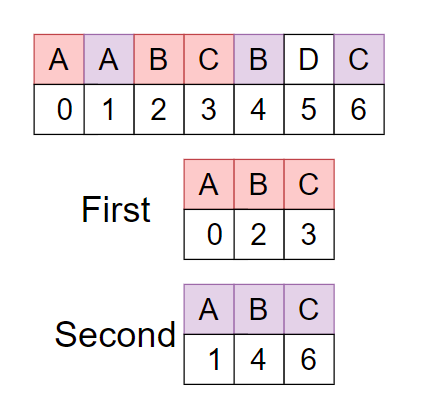# Longest Repeating Subsequence

Posted: 7 Feb, 2021
Difficulty: Moderate

## PROBLEM STATEMENT

#### You are given a string ‘st’, Your task is to find the length of the longest repeating subsequence such that two subsequences don’t have the same character at the same position.

##### For Example :
``````The given string st = AABCBDC.
````````````As you can see there are two repeating longest subsequences “ABC” with the same character but different position. Therefore the required answer is ‘3’ as the length of “ABC” is 3.
``````
##### Input Format :
``````The first line of input contains an integer ‘T’ denoting the number of test cases.

The first line of each test case contains a single integer ‘N’, where ‘N’ denotes the length of string ‘st’.

The second line of each test case contains a string ‘st’.
``````
##### Output Format :
``````For every test case, print the length of the longest repeating subsequence.

Output for each test case will be printed in a separate line.
``````
##### Note :
``````You do not need to print anything; it has already been taken care of. Just implement the given function.

All the characters of the given string ‘st’ are uppercase letters.
``````
##### Constraints :
``````1 <= T <= 50
1 <= N <= 100

Time Limit: 1 sec
``````Approach 1

The basic idea is the same as the longest common subsequence( LCS), only need to exclude the case when (i==j) because we can’t consider both same characters in both the subsequence.

LRS[i][j] = LRS[i-1][j-1] +1 where ( st[i-1] == st[j-1] and i!=j)

LRS[i][j] = max(LRS[i-1][j], LRS[i][j-1]) where ( st[i-1] != st[j-1)

Consider base case if(i==0 and j==0) then LRS=0

Algorithm

• LRSHelper( st , i, j), to return longest repeating subsequence
• if(i==0 or j==0 ), base case
• Return 0
• if( st[i-1] == st[j-1] and (i!=j) )
• Return LRS[i][j] = LRS[i-1][j-1] +1
• Else
• Return max(LRS[i-1][j], LRS[i][j-1])